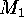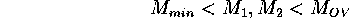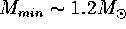Next: Case A: Up: No Title Previous: Introduction

# Mergingology

Let us assume that two neutron stars with massesandare merging. The following state of the after-merging object is determined by the ratio of the resulting total mass and the Oppenheimer-Volkoff limit. Two different scenarios may be distinguished as follows (Lipunova & Lipunov, 1998):Here and below we interpret the Oppenheimer-Volkoff limit not as the standard value derived for the cold equation of state of baryonic matter for a non-rotating neutron star, but as a modified one. In the general case the Oppenheimer-Volkoff limit is a function of the angular spin velocity of the object, its entropy, and the specific equation of state:.

Each neutron star can have a mass lying between the limits:-- derived by Landau (1938).-- commonly suggested now.

Lipunov V.M.
Fri Nov 28 17:12:56 MSK 1997